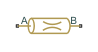# Hydraulic Resistive Tube

Hydraulic pipeline which accounts for friction losses only

## Library

Hydraulic Elements

•## Description

The Hydraulic Resistive Tube block models hydraulic pipelines with circular and noncircular cross sections and accounts for resistive property only. In other words, the block is developed with the basic assumption of the steady state fluid momentum conditions. Neither fluid compressibility nor fluid inertia is considered in the model, meaning that features such as water hammer cannot be investigated. If necessary, you can add fluid compressibility, fluid inertia, and other effects to your model using other blocks, thus producing a more comprehensive model.

The end effects are also not considered, assuming that the flow is fully developed along the entire pipe length. To account for local resistances, such as bends, fittings, inlet and outlet losses, and so on, all the resistances are converted into their equivalent lengths, and then the total length of all the resistances is added to the pipe geometrical length.

Pressure loss due to friction is computed with the Darcy equation, in which losses are proportional to the flow regime-dependable friction factor and the square of the flow rate. The friction factor in turbulent regime is determined with the Haaland approximation (see ). The friction factor during transition from laminar to turbulent regimes is determined with the linear interpolation between extreme points of the regimes. As a result of these assumptions, the tube is simulated according to the following equations:

`$p=f\frac{\left(L+{L}_{eq}\right)}{{D}_{H}}\frac{\rho }{2{A}^{2}}q\cdot |q|$`

`$\mathrm{Re}=\frac{q\cdot {D}_{H}}{A\cdot \nu }$`

where

 p Pressure loss along the pipe due to friction q Flow rate through the pipe Re Reynolds number ReL Maximum Reynolds number at laminar flow ReT Minimum Reynolds number at turbulent flow Ks Shape factor that characterizes the pipe cross section fL Friction factor at laminar border fT Friction factor at turbulent border A Pipe cross-sectional area DH Pipe hydraulic diameter L Pipe geometrical length Leq Aggregate equivalent length of local resistances r Height of the roughness on the pipe internal surface ν Fluid kinematic viscosity

The block positive direction is from port A to port B. This means that the flow rate is positive if it flows from A to B, and the pressure loss is determined as $p={p}_{A}-{p}_{B}$.

### Variables

To set the priority and initial target values for the block variables prior to simulation, use the Initial Targets section in the block dialog box or Property Inspector. For more information, see Set Priority and Initial Target for Block Variables.

Nominal values provide a way to specify the expected magnitude of a variable in a model. Using system scaling based on nominal values increases the simulation robustness. Nominal values can come from different sources, one of which is the Nominal Values section in the block dialog box or Property Inspector. For more information, see Modify Nominal Values for a Block Variable.

## Basic Assumptions and Limitations

• Flow is assumed to be fully developed along the pipe length.

• Fluid inertia, fluid compressibility, and wall compliance are not taken into account.

## Parameters

Tube cross section type

The type of tube cross section: `Circular` or `Noncircular`. For a circular tube, you specify its internal diameter. For a noncircular tube, you specify its hydraulic diameter and tube cross-sectional area. The default value of the parameter is `Circular`.

Tube internal diameter

Tube internal diameter. The parameter is used if Tube cross section type is set to `Circular`. The default value is `0.01` m.

Noncircular tube cross-sectional area

Tube cross-sectional area. The parameter is used if Tube cross section type is set to `Noncircular`. The default value is `1e-4` m^2.

Noncircular tube hydraulic diameter

Hydraulic diameter of the tube cross section. The parameter is used if Tube cross section type is set to `Noncircular`. The default value is `0.0112` m.

Geometrical shape factor

Used for computing friction factor at laminar flow. The shape of the tube cross section determines the value. For a tube with a noncircular cross section, set the factor to an appropriate value, for example, 56 for a square, 96 for concentric annulus, 62 for rectangle (2:1), and so on . The default value is `64`, which corresponds to a tube with a circular cross section.

Tube length

Tube geometrical length. The default value is `5` m.

Aggregate equivalent length of local resistances

This parameter represents total equivalent length of all local resistances associated with the tube. You can account for the pressure loss caused by local resistances, such as bends, fittings, armature, inlet/outlet losses, and so on, by adding to the pipe geometrical length an aggregate equivalent length of all the local resistances. The default value is `1` m.

Internal surface roughness height

Roughness height on the tube internal surface. The parameter is typically provided in data sheets or manufacturer’s catalogs. The default value is `1.5e-5` m, which corresponds to drawn tubing.

Laminar flow upper margin

Specifies the Reynolds number at which the laminar flow regime is assumed to start converting into turbulent. Mathematically, this is the maximum Reynolds number at fully developed laminar flow. The default value is `2000`.

Turbulent flow lower margin

Specifies the Reynolds number at which the turbulent flow regime is assumed to be fully developed. Mathematically, this is the minimum Reynolds number at turbulent flow. The default value is `4000`.

## Global Parameters

Parameters determined by the type of working fluid:

• Fluid density

• Fluid kinematic viscosity

Use the Hydraulic Fluid (Simscape Fluids) block or the Custom Hydraulic Fluid block to specify the fluid properties.

## Ports

The block has the following ports:

`A`

Hydraulic conserving port associated with the tube inlet.

`B`

Hydraulic conserving port associated with the tube outlet.

## References

 White, F.M., Viscous Fluid Flow, McGraw-Hill, 1991

## Version History

Introduced in R2009b